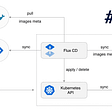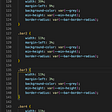# Day#4 [ 21 Days — C Programming ]

After learning input and output now we will learn some operator in C .

What are Operator ?

Operator performs operation on variables .

Why we need operator ?

We need operator to perform operation on variables like adding , multiplying or checking two variables relation or performing bit operation on them or to modify value etc.

Types of Operator :

1. Arithmetic Operator
2. Logical Operator
3. Bitwise Operator
4. Relational Operator
5. Assignment Operator
6. Conditional Operator
7. Special Operator

1. Arithmetic Operator

Arithmetic Operator performs arithmetic operation like addition, subtraction, multiplication, division and remainder.

• - ( Subtraction )
• * ( Multiplication )
• / ( Division )
• % ( Remainder )

2. Logical Operator

• && ( AND Operator )
• || ( Or Operator )
• ! ( Not Operator )

&& ( AND ) Table :

* Both are True then only result is True.

True && True = True

True && False = False

False && True = False

False && False = False

|| ( OR ) Table :

* Both are False then only result is False.

True || True = True

True || False = True

False || True = True

False || False = False

! ( NOT Table )

!True = False

!False = True

* Non-zero is considered as True and Zero is considered as False in C Programming.

3. Bitwise Operator

• & ( Bitwise AND Operator )
• | ( Bitwise OR Operator )
• ~ ( Bitwise NOT Operator )
• ^ ( Bitwise XOR Operator )

& ( Bitwise AND ) Table :

1 & 1 = 1

1 & 0 = 0

0 & 1= 0

0 & 0= 0

& ( Bitwise OR ) Table :

1 | 1 = 1

1 | 0 = 1

0 | 1= 1

0 | 0= 0

~ ( Bitwise NOT ) Table :

~1 = 0

~0 = 1

^ ( Bitwise XOR ) Table :

* If Both are same Result is 0 , if Both are different Result is 1.

1 ^ 1 = 0

1 ^ 0 = 1

0 ^ 1= 1

0 ^ 0= 0

4. Relational Operator

• == ( Equal )
• != ( Not Equal )
• < ( Less than )
• > ( Greater than )
• <= ( Less than or Equal to )
• >= ( Greater than or Equal to )

5. Assignment Operator

• = ( Assignment )
• += ( Addition Assignment )
• -= ( Subtraction Assignment )
• *= ( Multiplication Assignment )
• /= ( Division Assignment )
• %= ( Remainder Assignment )
• ++ ( Unary - Increment Operator )
• -- ( Unary - Decrement Operator )

Let’s Connect with us :

Instagram : https://www.instagram.com/vikasss_7663/

--

--

--

## More from Vikas Shekhawat

Software engineer and a happy 😊 person .

Love podcasts or audiobooks? Learn on the go with our new app.

## Git Merge vs Git Rebase## Modernizing Tidepool: Part 3: Continuous Delivery with GitOps## API Modernization using Apigee## Running Kafka and KSQL on your Raspberry Pi 4## Displaying objects on maps in the Django administration site.## How can you, a business analyst ensure smooth software handover## Vikas Shekhawat

Software engineer and a happy 😊 person .

## Why Object-Oriented Programming OOPs?## GCC and the steps of compilation## Pointer Arithmetic In C++